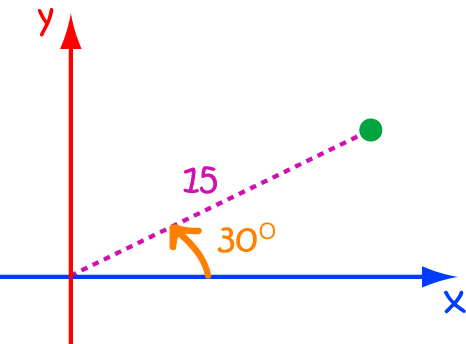# Definition of Polar CoordinatesPolar coordinates give us a way to locate a point in the plane, by specifying its distance $r$ from the origin, and the angle a line from the origin through the point makes with the horizontal.

Polar coordinates can be written as the ordered pair $(r,\theta)$, where $r$ is the distance from the origin, and $\theta$ is the angle the line through the point makes with the horizontal axis.

For example, the point shown in the picture would have polar coordinates $(15,30^\circ)$.

### Description

The aim of this dictionary is to provide definitions to common mathematical terms. Students learn a new math skill every week at school, sometimes just before they start a new skill, if they want to look at what a specific term means, this is where this dictionary will become handy and a go-to guide for a student.

### Audience

Year 1 to Year 12 students

### Learning Objectives

Learn common math terms starting with letter P

Author: Subject Coach
You must be logged in as Student to ask a Question.